# SVM实现邮件分类

Posted by jjx on December 16, 2016

• Training Linear SVM
• Implementing Gaussian Kernel
• Training SVM with RBF Kernel
• 选择最优的C, sigma参数
• 画出边界线

C = 1;
model = svmTrain(X, y, C, @linearKernel, 1e-3, 20);
visualizeBoundaryLinear(X, y, model);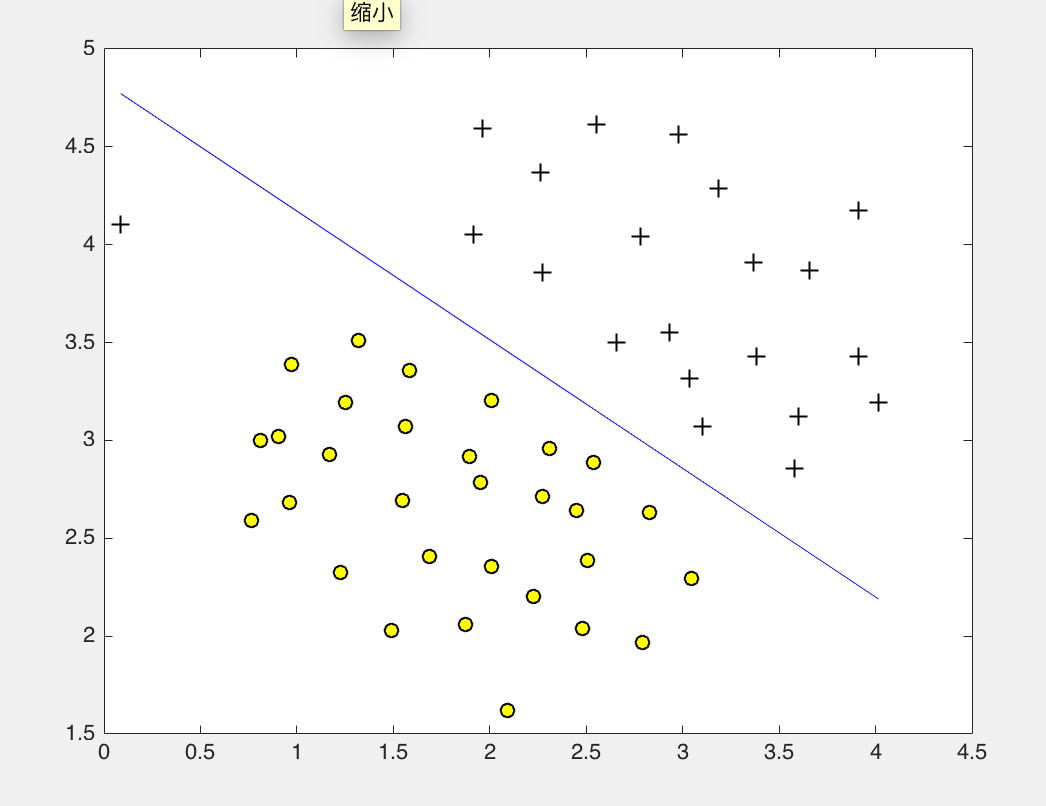function sim = gaussianKernel(x1, x2, sigma)
x1 = x1(:); x2 = x2(:);
sim = 0;
sim = exp( - (x1-x2)'* (x1-x2) / (2 * sigma *sigma ) );
end

% SVM Parameters
C = 1; sigma = 0.1;

% We set the tolerance and max_passes lower here so that the code will run
% faster. However, in practice, you will want to run the training to
% convergence.
model= svmTrain(X, y, C, @(x1, x2) gaussianKernel(x1, x2, sigma));
visualizeBoundary(X, y, model);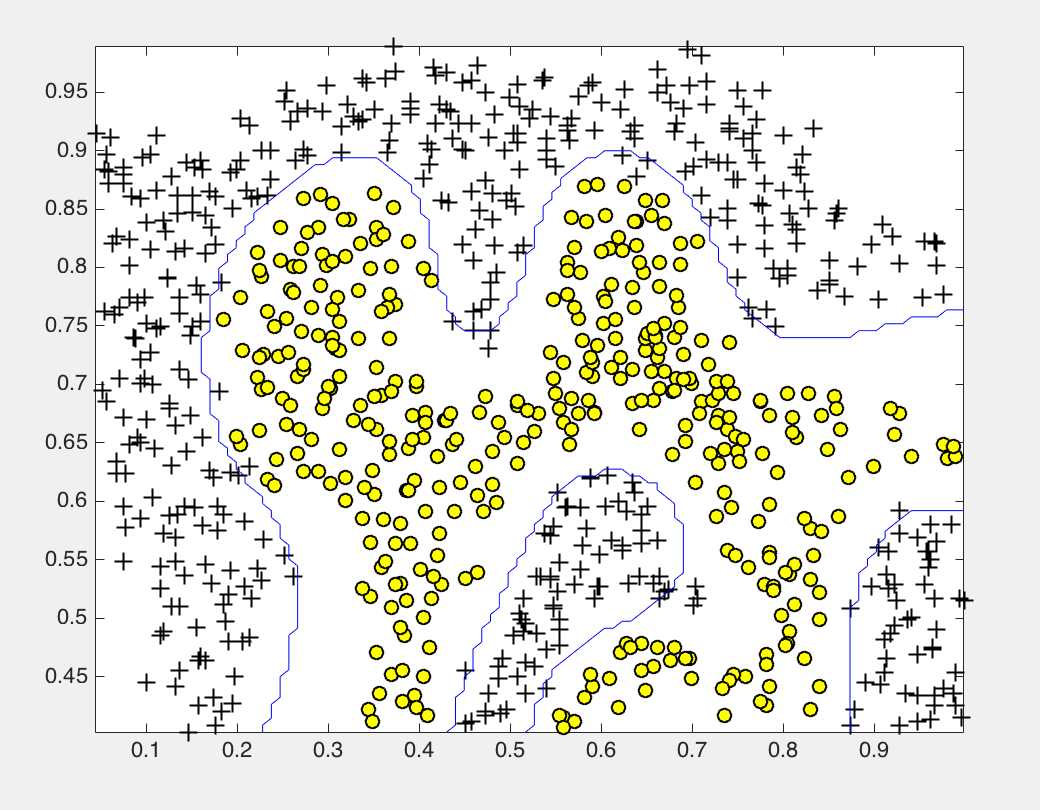function [C, sigma] = dataset3Params(X, y, Xval, yval)
C = 1;
sigma = 0.3;
C_vec = [0.01 0.03 0.1 0.3 1 3 10 30]';
sigma_vec = [0.01 0.03 0.1 0.3 1 3 10 30]';
error_val = zeros(length(C_vec),length(sigma_vec));
error_train = zeros(length(C_vec),length(sigma_vec));
for i = 1:length(C_vec)
for j = 1:length(sigma_vec)
model= svmTrain(X, y, C_vec(i), @(x1, x2) gaussianKernel(x1, x2, sigma_vec(j)));
predictions = svmPredict(model, Xval);
error_val(i,j) = mean(double(predictions ~= yval));
end
end
% figure
% error_val
% surf(C_vec,sigma_vec,error_val)   % 画出三维图找最低点

[minval,ind] = min(error_val(:));   % 0.03
[I,J] = ind2sub([size(error_val,1) size(error_val,2)],ind);
C = C_vec(I)         %   1
sigma = sigma_vec(J)  %   0.100

% [I,J]=find(error_val ==  min(error_val(:)) );    % 另一种方式找最小元素位子
% C = C_vec(I)         % 1
% sigma = sigma_vec(J)  % 0.100
end

[C, sigma] = dataset3Params(X, y, Xval, yval);

% Train the SVM
model= svmTrain(X, y, C, @(x1, x2) gaussianKernel(x1, x2, sigma));
visualizeBoundary(X, y, model);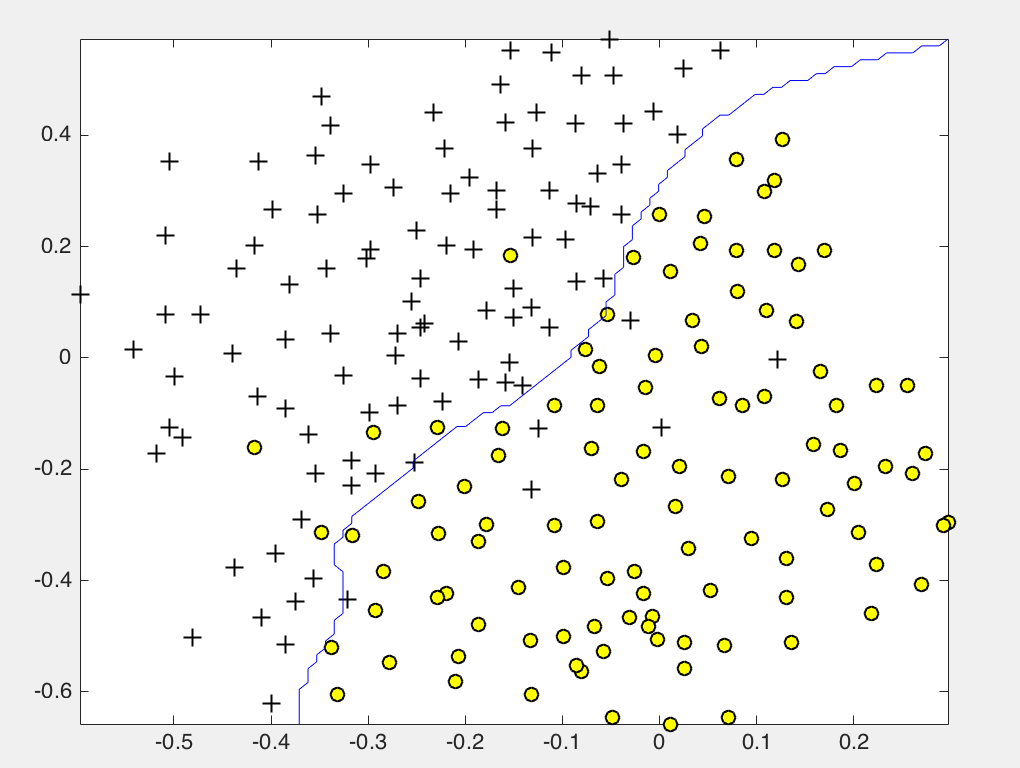#### 邮件分类

• 邮件数据归一化处理
• 特征提取
• Train Linear SVM for Spam Classification
• Test Spam Classification
• Top Predictors of Spam
• 测试自己的email

In processEmail.m, we have implemented the following email prepro- cessing and normalization steps:

• Lower-casing: The entire email is converted into lower case, so that captialization is ignored (e.g., IndIcaTE is treated the same as Indicate).
• Stripping HTML: All HTML tags are removed from the emails. Many emails often come with HTML formatting; we remove all the HTML tags, so that only the content remains.
• Normalizing URLs: All URLs are replaced with the text “httpaddr”.
• Normalizing Numbers: All numbers are replaced with the text “number”.
• Normalizing Dollars: All dollar signs (\$) are replaced with the text “dollar”.
• Word Stemming: Words are reduced to their stemmed form. For ex- ample, “discount”, “discounts”, “discounted” and “discounting” are all replaced with “discount”. Sometimes, the Stemmer actually strips off additional characters from the end, so “include”, “includes”, “included”, and “including” are all replaced with “includ”.
• Removal of non-words: Non-words and punctuation have been re- moved. All white spaces (tabs, newlines, spaces) have all been trimmed to a single space character.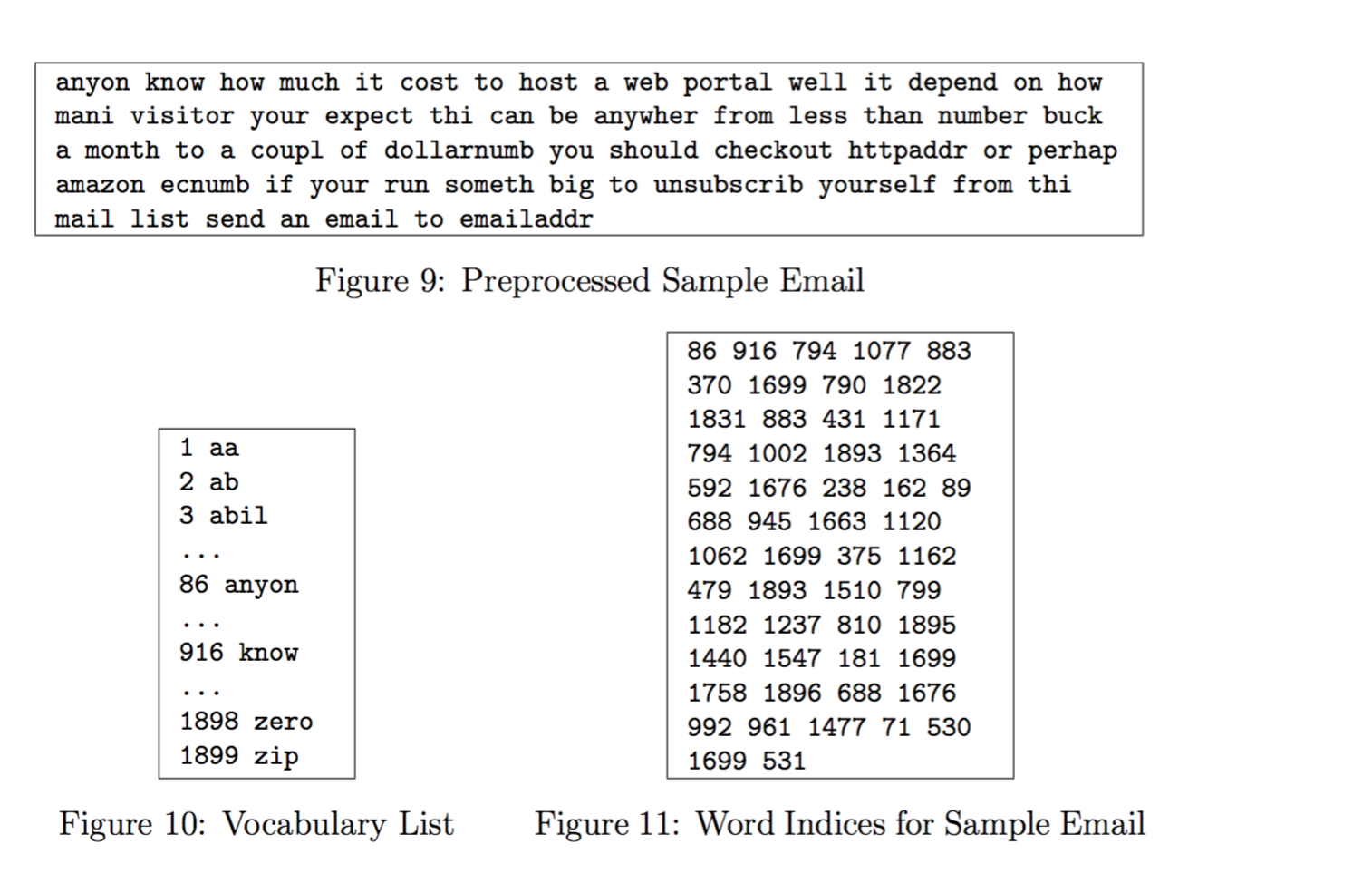Vocabulary List

Our vocabulary list was selected by choosing all words which occur at least a 100 times in the spam corpus, resulting in a list of 1899 words. In practice, a vocabulary list with about 10,000 to 50,000 words is often used.

    for i=1:length(vocabList)
if( strcmp(vocabList{i}, str) )
word_indices = [word_indices;i];
end
end


Extracting Features from Emails

You should look up the word in the vocabulary list vocabList and find if the word exists in the vocabulary list. If the word exists, you should add the index of the word into the word indices variable. If the word does not exist, and is therefore not in the vocabulary, you can skip the word.

function x = emailFeatures(word_indices)
% Total number of words in the dictionary
n = 1899;

% You need to return the following variables correctly.
x = zeros(n, 1);
x(word_indices) = 1;
end


Training SVM for Spam Classification

load('spamTrain.mat');

fprintf('\nTraining Linear SVM (Spam Classification)\n')
fprintf('(this may take 1 to 2 minutes) ...\n')

C = 0.1;
model = svmTrain(X, y, C, @linearKernel);

p = svmPredict(model, X);

fprintf('Training Accuracy: %f\n', mean(double(p == y)) * 100);

%% =================== Part 4: Test Spam Classification ================

fprintf('\nEvaluating the trained Linear SVM on a test set ...\n')

p = svmPredict(model, Xtest);

fprintf('Test Accuracy: %f\n', mean(double(p == ytest)) * 100);


After loading the dataset, ex6 spam.m will proceed to train a SVM to classify between spam (y = 1) and non-spam (y = 0) emails. Once the training completes, you should see that the classifier gets a training accuracy of about 99.8% and a test accuracy of about 98.5%.

Top Predictors for Spam

[weight, idx] = sort(model.w, 'descend');
vocabList = getVocabList();

fprintf('\nTop predictors of spam: \n');
for i = 1:15
fprintf(' %-15s (%f) \n', vocabList{idx(i)}, weight(i));
end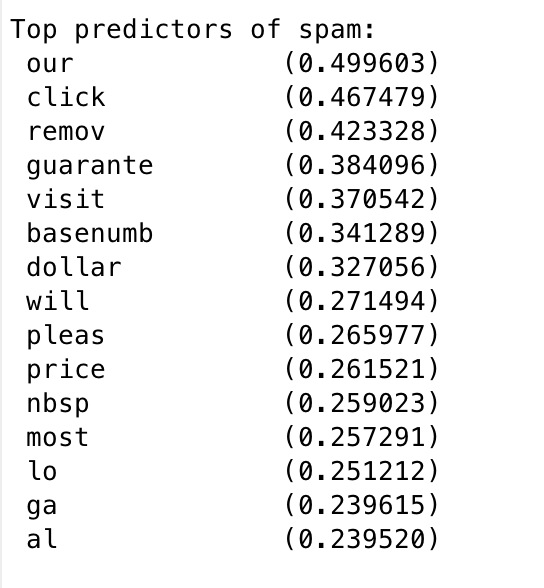filename = 'spamSample1.txt';